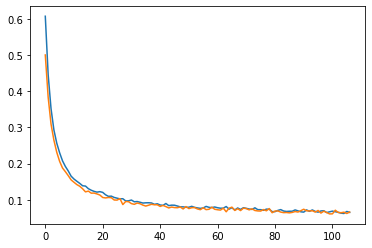# Loss is low, but all the prediction near 0.5 for binary classification

I am having a binary classification task.
My network

``````def set_parameter_requires_grad(model, feature_extracting):
if feature_extracting:
for param in model.parameters():

model = models.resnet18(pretrained=True)

model.fc = nn.Sequential(nn.Linear(in_features=512, out_features=1, bias=True))
``````

loss vs epochs curve(orange is validation loss)But when I get the predictions, all the outputs are near 0.5 (which is like the model was not trained at all)
Since the loss function used was nn.BCEWithLogitsLoss(), I added an explicit sigmoid while generating the predictions.
The way I generate the predictions

``````transformed = data_transforms(pil_img)
label = model(transformed.float().to(device).unsqueeze(0))
label = sigmoid(label.cpu().detach().numpy())
``````

Hi Satinder!

As an aside, if you train with `BCEWithLogitsLoss`, you will be training
your model to produce logits. A model making “neutral” predictions
will produce logits near `0.0` (that correspond to probabilities near `0.5`).

up.

The graph you posted shows your loss function falling below `0.1`.

A neutral prediction (a probability of `0.5` with `BCELoss` or a logit of
`0.0` with `BCEWithLogitsLoss`) will give a loss of `log (2)`, regardless
of the target value.

``````>>> tone = torch.ones (1)
>>> torch.nn.functional.binary_cross_entropy (0.5 * tone, tone)
tensor(0.6931)
>>> torch.nn.functional.binary_cross_entropy_with_logits (0.0 * tone, tone)
tensor(0.6931)
``````

(To get a loss of `0.1` you have to be pretty far away from neutral.)

So either the predictions underlying the training and validations losses
shown in the chart you posted aren’t neutral, or your loss calculation is
something different than the simple:

``````loss = torch.nn.BCEWithLogitsLoss() (predictions, targets)
``````

Best.

K. Frank